Self-Gravitating Stability of a Fluid Cylinder Embedded in a Bounded Liquid, Pervaded by Magnetic Field, for all Symmetric and Asymmetric Perturbation Modes
Journal of Biosensors & Bioelectronics

Like us on:

# Self-Gravitating Stability of a Fluid Cylinder Embedded in a Bounded Liquid, Pervaded by Magnetic Field, for all Symmetric and Asymmetric Perturbation Modes

Hamdy M Barakat*

Department of Mathematics, Faculty of Science and Arts, Al Jouf University, Al Jouf, The Kingdom of Saudi Arabia

*Corresponding Author:
Hamdy M Barakat
Department of Mathematics
Faculty of Science and Arts
Al Jouf University, Al Jouf
The Kingdom of Saudi Arabia
Tel: +96646257869
E-mail: [email protected]

Received Date: December 19, 2016; Accepted Date: December 27, 2016; Published Date: December 30, 2016

Citation: Barakat HM (2016) Self-Gravitating Stability of a Fluid Cylinder Embedded in a Bounded Liquid, Pervaded by Magnetic Field, for all Symmetric and Asymmetric Perturbation Modes. J Biosens Bioelectron 7:234. doi: 10.4172/2155- 6210.1000234

Copyright: © 2016 Barakat HM. This is an open-access article distributed under the terms of the Creative Commons Attribution License, which permits unrestricted use, distribution, and reproduction in any medium, provided the original author and source are credited.

Visit for more related articles at Journal of Biosensors & Bioelectronics

#### Abstract

The self-gravitating stability of a fluid cylinder embedded in a bounded liquid, pervaded by magnetic field, for all symmetric and asymmetric perturbation modes has been discussed. The problem is formulated and the (MHD) basic equations are solved A general eigen-value relation is derived studied analytically and results are confirmed numerically. The stability of a fluid cylinder under the action of self-gravitating, inertia, and electromagnetic forces is developed. The electromagnetic force has stabilizing and destabilizing influences in the axisymmetric modes. For very high intensity of magnetic field the model is completely stable for all values of wavelengths. This phenomenon is interest, academically and during the geological drilling in the crust of the earth as we have superposed gas-oil layer mixture fluids. The stability behavior of the model comes after destabilizing behavior of the model when it be reduced and suppressed.

#### Keywords

Hydromagnetic stability: Self-gravitating; Electromagnetic force

#### Introduction

The object of the present work is to investigate the hydro-magnetic (MHD) stability of annular fluid jet. The MHD stability of full fluid cylinder pervaded by uniform magnetic field has been documented by Chandrasekhar . Kendall performed experiments to obtain and examine the stability of annular fluid jet. Moreover, he did attract and draw the attention for investigating the stability of this model in general for its crucial astrophysical applications. The classical of the capillary instability of a gas cylinder submerged into a liquid are given for first time by Chandrasekhar for axisymmetric perturbation . Hasan and Abdelkhalek, Elazab et al., and Drazin and Reid gave the dispersion relation valid for all axisymmetric and non-ax symmetric modes [2-4]. Cheng discussed the instability of a gas jet in an incompressible liquid for all modes of perturbation. However, we have to mention here that the results given by Cheng . Kendall  performed experiments with modern equipment to check the breaking up of that model. Barakat HM study the magneto hydrodynamic (MHD) Stability of Oscillating Fluid Cylinder with Magnetic Field . Barakat  discuss the axisymmetric magneto-hydrodynamic (MHD) self-gravitating stability of fluid cylinder. Mehring and Sirignano  discuss the axisymmetric capillary waves on thin annular liquid sheets. The aim of the present study the self-gravitating stability of a fluid cylinder embedded in a bounded liquid, pervaded by magnetic field, for all symmetric and asymmetric perturbation modes.

Formulation of the problem

Consider a gas cylinder of radius (a) surrounded by a bounded liquid of cylindrical shape of radius (qa) where 1<q<∞. Both of the two fluids are pervaded by a uniform magnetic field H0=(0, 0, α H0). The density of the gas is assumed to be ρ(1) while that of the bounded liquid is ρ(2). The gas and the liquid are assumed to be incompressible, non-viscous, perfectly conducting and streaming with velocity U0=(0, 0, U). This bounded gas-core liquid jet surrounded by a self-gravitating medium. Each of the two fluids is acting upon by the self-gravitating, electromagnetic field and it is pressure gradient force. Surrounding medium is acted upon by self-gravitating force only. For the problem at hand these equations are given by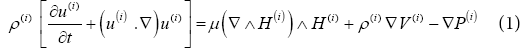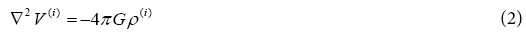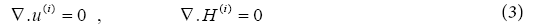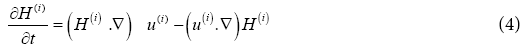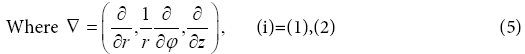In the medium surrounding the gas-core bounded liquid, we have

2 V(i)=0 (6)

The gas medium superscript (1), while for the liquid have superscript (2).

Where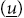and (P) are the fluid velocity vector and kinetic pressure respectively,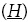is the magnetic field intensity, (V) the self-gravitating potential and (G) the self-gravitating constant. The system of basic equation (1)- (6) are solved for the unperturbed state. Consequently, the relation relating the magnetic, surface and fluids kinetic pressures is given by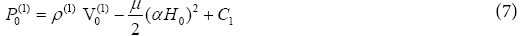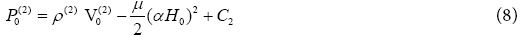Applying the balance of the pressure across the fluid interface r=a, and r=qa, we find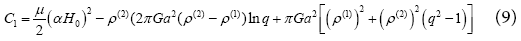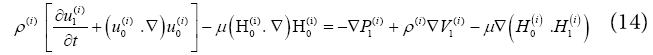Perturbation analysis

For small departure from the unperturbed state, every physical quantity Q(r,φ,z,t) could be expressed as

Q(r,φ,z,t)=Q0(r)+ε0(t) Q1(r,φ,z)+? (11)

Where Q stands for u(i), P(i), H(i), V(i) the amplitude of perturbation ε(t) at time t is

ε(t)=ε0exp(σ t) (12)

Where σ is the growth rate of the instability or rather the oscillation frequency if (σ=iw with i= √(-1)) is imaginary. The perturbed radii distances f the gas cylinder is given by

r=a+ε0a1 where a1=a exp(σt+i(km+mφ)) (13)

Where (k) is the longitudinal wave number and (m an integer) is the transverse wave number. The linearized perturbation equation deduced from the fundamental equations (1) - (7) are given by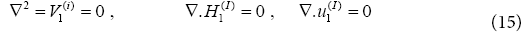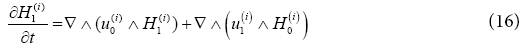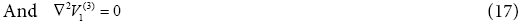Based on the linear perturbation technique and stability theory, every perturbed quantity Q1 (r,φ,z,t) could be expressed as exp(σt+i(km+mφ)) times an amplitude function of r,

Q1 (r, ?, z, t) = q1 (r)exp (σ t + i (km + m?)) (18)

By an apple to expansion (18), the relevant perturbation equation (14)-(17) are solved.

Finally, the non-singular solution is given by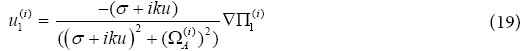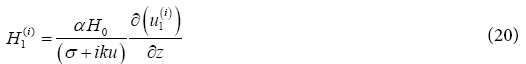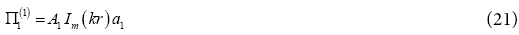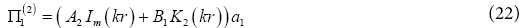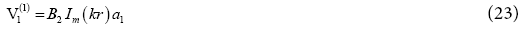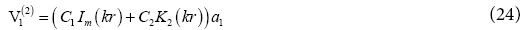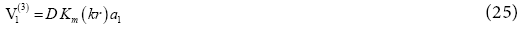Where Im(kr) and Km(kr) are the modified Bessel function of first and second kind of order m, a while A1, A2, B1, B2, C1, C2, and D are constant of integration to be determined by the appropriate boundary condition and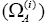is the Alfven wave frequency defined in terms of H0 by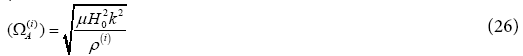Boundary condition

The solutions of equation for the unperturbed system and perturbed system must satisfy certain boundary condition across the fluid interface at (r=a, and r=qa). These boundary conditions are given as follows:

1) The normal component of the velocity vector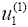must be compatible with the velocity of the gas-liquid interface particles across the surface at (r=a) i.e.,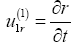2) The radial component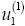of the gas velocity vector u(1) must equal that of the liquid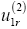at r=a i.e.,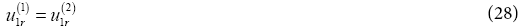3) The normal componentof the velocity vector u(2) of the liquid region vanishes across the liquid-tenuous medium at (r=qa) i.e.,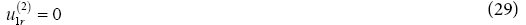4) The self-gravitating potential of the gas and the liquid and their derivatives are continuous across the gas-liquid interface at (r=a) i.e.,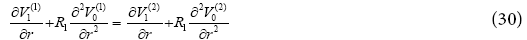And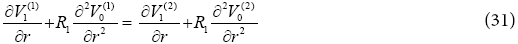5) The self-gravitating potential of the liquid and tenuous medium and their derivatives must be continuous at (r=qa)

6) The normal component of the magnetic field must be continuous across the gas-liquid interface at (r=a)

Upon applying the foregoing boundary-condition at (r=a) and (r=qa), the constant of integration are identified as follows: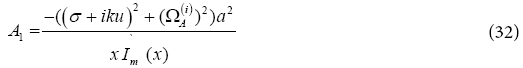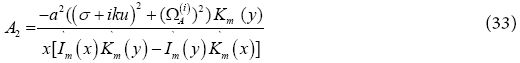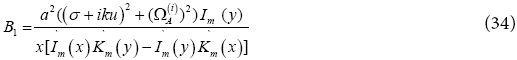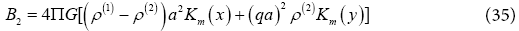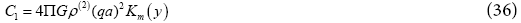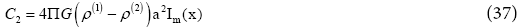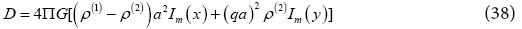Where (x=ka and y=qx) are the dimensionless longitudinal wave numbers.

By resorting to the foregoing solution of equations for the unperturbed and perturbed state and by applying the compatibility condition, that the normal component of the total stress must be continuous across the gas-liquid interface at (r=a), the following stability criterion can be derived state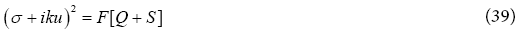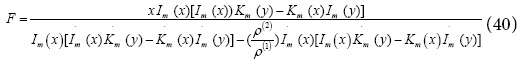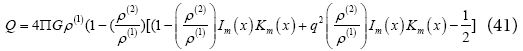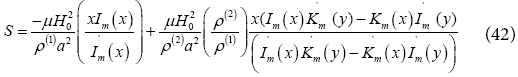#### Discussion

Equation (39) is the desired relation of the present model of a gas cylinder volume embedded into a bounded liquid subjected to a selfgravitating, pressure-gradient and magneto-dynamic force. It relates the growth rate σ with the modified Bessel function I0(x) and K0(x) and their derivative, the wave number m and x, the amplitude U of the streaming velocity, Ω the oscillation frequency of the oscillating streaming, α is the parameter of the magnetic field in the gas cylinder and with the parameters T, ρ, a, μ and H0 of the problem. One has to mention here that the relation (39) contain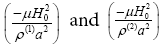as a unit of (time)-2. The denisities (ρ(1) , ρ(2)) of the gas and liquid region, the gas-liquid radii ratio q, the magnetic field intensity and permeability H0 and μ, the self-gravitating constant G for the gas radius a. The dispersion relation (39) is the linear combination of a of a dispersion relation of the same model being acted upon by the self-gravitating force only. In the latter work, Chandrasekhar utilized the technique of presenting the solenoidal vectors in terms of poloidal and toroidal quantities which are valid only for the m=0. This linear combination is also true if the acting force is the capillary and electromagnetic force whether the model is full fluid cylinder .

Stability discussion

Before we discuss the ordinary stability, marginal stability and instability of the system under consideration, it is desirable to study the behaviors of the Bessel functions and also those of the compound functions contained in the relation (39).

In view of the recurrence relations (see Abramowitz and Stegun  )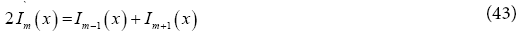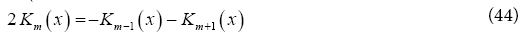Because Im(x) is monotonic increasing and positive definite Im(x) > 0 for all modes of perturbation m ≥ 0 and nonzero values of x ≠ 0, while Km(x) is monotonically decreasing but never negative, i.e., Km(x) > 0 we may show that

I ?m(x) > 0, Km ?(x) < 0 (45)

Also for, m ≥ 1 for all values of x ≠ 0, we have

2Im(x) Km(x) < 1 (46)

In order to discuss the stability and instability states region of the present models, the dispersion relation (39). One has to refer here that if we suppose that (m=0) the dispersion relation yields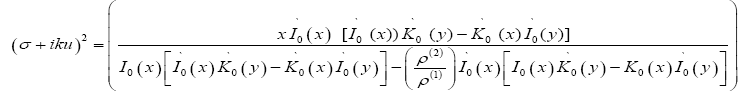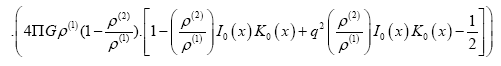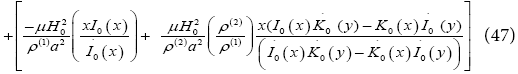And we use the relation

K0 ?(x)=-K1(x), I0 ?(x)=I1(x) (48)

With the Wronskian relation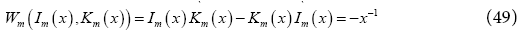The dispersion relation (39) reduce to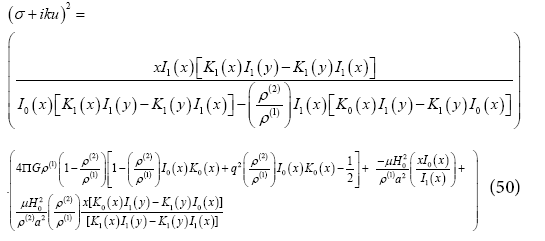Limiting cases

Some previous reported works could be obtained as limiting cases for the present general dispersion relation (41). A lot of approximation (ρ(2)=0, Ho=0, u=0 and m=0) are required for the criterion (52), to obtain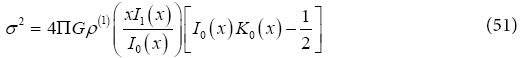The relation (52) was derived for the first time by Chandrasekhar and Fermi  using a different technique from that which is used here.

If we assume that (ρ(2)=0, Ho=0, u=0 and m ≥ 0)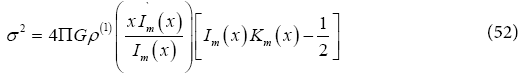This corroborates the relation derived by Chandrasekhar  using the normal-mode analysis. If we suppose that the fluids are not conducting and (H0=0) we obtain from (39)

(σ + iku)2 = F . M (53)

Where F and M are defined by equations (40), (41). Equation (53) is the dispersion relations for the present model under the effect only the self-gravitating force in the gas and liquid. If we suppose that t (G=0) we obtain from (39)

(σ + iku)2 = F . M (53)

Where F and S are defined by equations (40), (43). Equation (54) is the dispersion relations for the present model under the effect only the electromagnetic force due to the existence of the uniform magnetic field in the gas and liquid .

#### Conclusion

1- The self-gravitating force is destabilizing only in the symmetric mode (m=0) for a small range of wave numbers, but it is stabilizing for all other perturbation.

2- The electromagnetic force due to the pervading uniform magnetic field in the gas and the liquid wave a strong stabilizing influence for all short and long wavelengths in all symmetric and asymmetric modes of perturbation.

3- The liquid-gas radii ratio has stabilizing tendency and that is valid for all x ≠ 0 and m ≥ 0 values.

4- The liquid-gas densities ratio a strong destabilizing effect for all wavelengths in all the symmetric and asymmetric modes of perturbation.

#### References

Select your language of interest to view the total content in your interested language

### Article Usage

• Total views: 925
• [From(publication date):
December-2016 - Jan 24, 2020]
• Breakdown by view type
• HTML page views : 829Can't read the image? click here to refresh